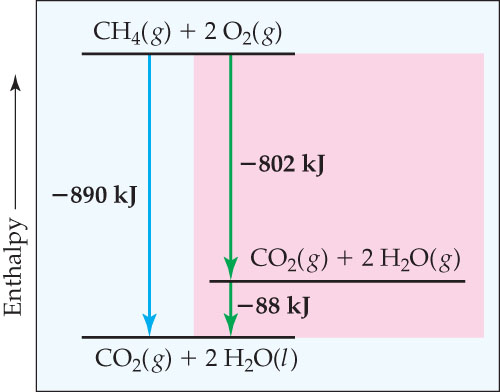# Problem: Enthalpy diagram for combustion of 1 mol of methane. The enthalpy change of the one-step reaction equals the sum of the enthalpy changes of the reaction run in two steps: -890 kJ = -802 kJ + (-88 kJ).What process corresponds to the -88 kJ enthalpy change?

###### FREE Expert Solution
85% (115 ratings)###### Problem DetailsEnthalpy diagram for combustion of 1 mol of methane. The enthalpy change of the one-step reaction equals the sum of the enthalpy changes of the reaction run in two steps: -890 kJ = -802 kJ + (-88 kJ).

What process corresponds to the -88 kJ enthalpy change?

Frequently Asked Questions

What scientific concept do you need to know in order to solve this problem?

Our tutors have indicated that to solve this problem you will need to apply the Enthalpy of Formation concept. You can view video lessons to learn Enthalpy of Formation. Or if you need more Enthalpy of Formation practice, you can also practice Enthalpy of Formation practice problems.

What professor is this problem relevant for?

Based on our data, we think this problem is relevant for Professor Deon's class at University of Colorado at Colorado Springs.